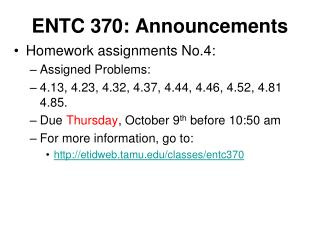DownloadDownload PresentationENTC 370: Announcements

# ENTC 370: Announcements

Télécharger la présentation## ENTC 370: Announcements

- - - - - - - - - - - - - - - - - - - - - - - - - - - E N D - - - - - - - - - - - - - - - - - - - - - - - - - - -
##### Presentation Transcript

1. ENTC 370: Announcements • Homework assignments No.4: • Assigned Problems: • 4.13, 4.23, 4.32, 4.37, 4.44, 4.46, 4.52, 4.81 4.85. • Due Thursday, October 9th before 10:50 am • For more information, go to: • http://etidweb.tamu.edu/classes/entc370

2. ENTC 370: Announcements • Exam I: • Thursday, Oct 23rd • Chapters 1 – 5 • Homeworks1 – 5 • Closed book/closed notes • Students will be allowed to bring own equation sheet • Double-sided is ok • Size: 8½ x 11

3. Specific Heat • Indicator of energy storage capabilities of various substances (physical property) • Defined as the energy required to raise the temperature of a unit mass of a substance by one degree • Two types: • Specific Heat at constant volume, CV • Specific Heat at constant pressure, CP

4. These are property relations or independent of the type of process Valid for any substance Units: kJ/kg-K or kJ/kg-° C Subscripts v and P stand for constant specific volume and constant pressure, respectively. Formal Definitions of Specific Heat

5. Internal Energy (u), Enthalpy (h) and Specific Heats (cp & cv) of Ideal Gases • For gases, u and h only depends on Temperature: u = f(T) In the case of air: Use Table A-17

6. Example For 5 min • Determine the final temperature, T2 • Identify type of fluid, type of device, energy flows • What remains constant? • Write down 1st Law and solve for unknown (T2)

7. Example Mass of piston is such that a 350 kPa pressure is required to move it. http://brod.sfsb.hr/test/testhome/vtAnimations/animations/chapter05/closed/processes/uniform/index.html Heat is added until the volume doubles. Find final temperature, work done by the air and total heat transferred to air.

8. Internal Energy, Enthalpy and Specific Heats of Solids and Liquids • Solids and liquids are incompressible substances, r ≠ f(Pressure); density remains rather constant regardless of pressure • CP = CV = C ← Good approximation for small changes in temperature

9. Internal Energy, Enthalpy and Specific Heats of Solids and Liquids • For solids • For liquids • Constant Pressure Process • Constant Temperature Process

10. Example • A 50 kg iron block at 80 °C is dropped into an insulated tank that contains 0.5 m3 of liquid water at 25 °C. Determine the temperature when thermal equilibrium is reached. • Identify material type (solid, liquid, etc.) • Write down 1st law for the described system • Solve for unknown

11. Chapter 5: Mass and Energy Analysis of Control Volumes (Open Systems) Conservation of Mass Principle • Continuity for any fluid (gas or liquid) • Mass flow rate In = Mass Flow Rate out • r1*A1*v1 = r2*A2*v2 • Continuity for liquids (incompressible flow) • A1*v1 = A2*v2

12. Mass Flow Rates Mass flow rate for any open system: Mass flow rate: The average velocity Vavg is defined as the average speed through a cross section.

13. Volume Flow Rates Definition of average velocity: Volume flow rate: The volume flow rate is the volume of fluid flowing through a cross section per unit time. The average velocity Vavg is defined as the average speed through a cross section.

14. Conservation of Mass Principle Mass In – Mass Out = Net Change in Mass within system

15. Conservation of Mass Principle The conservation of mass principle for a control volume: The net mass transfer to or from a control volume during a time interval t is equal to the net change (increase or decrease) in the total mass within the control volume during t.

16. Conservation of Mass for General Control Volume The conservation of mass principle for the open system or control volume is expressed as

17. Conservation of Mass Principle General conservation of mass: General conservation of mass in rate form: or

18. Control surface Control Volume Mass in system, m(t) Mass flow into control surface, kg/s An unsteady situation: dmC.V./dt >0

19. A steady situation: dmC.V./dt =0 Control surface Control Volume Mass outflow Mass inflow

20. Steady Flow Process During a steady-flow process, the total amount of mass contained within a control volume does not change with time (mCV = constant). Then the conservation of mass principle requires thatthe total amount of mass entering a control volume equal the total amount of mass leaving it.

21. Steady-state, Steady-Flow Conservation of Mass Since the mass of the control volume is constant with time during the steady-state, steady-flow process, the conservation of mass principle becomes

22. Flow Work • P=F/A (definition of pressure) • F = P*A (finding force using pressure) • Work = Force*Distance • Work = F*L = P*A*L = P*Volume • Work/mass = P*v

23. Total Energy of Simple Compressible System Consists of three parts without flow work: Internal Energy Kinetic Energy Potential Energy